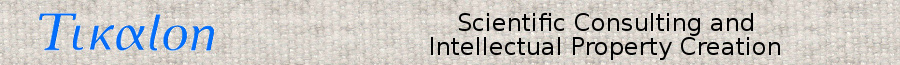Squaring the Circle

September 23, 2019

I was fascinated by the Pythagorean theorem as an elementary school student, principally because it was a bit of fundamental mathematics that was accessible to someone at my educational level. This theorem is traditionally ascribed to the Greek philosopher, Pythagoras, who, like most of the Pre-Socratic philosophers, followed in the tradition of Thales. Thales rejected the idea that things happened at the whim of the gods and sought scientific explanations of natural phenomena. Thales is famous for Thales' theorem, that any angle inscribed in a semicircle is a right angle.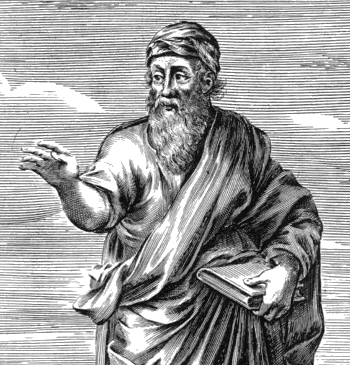Pythagoras (Πυθαγορας) lived on the Greek island of Samos from about 570 BC - 495 BC. Pythagoras was a Pre-Socratic philosopher and the founder of a religious movement that considered numbers to be the fundamental reality, a philosophy that string theorists find comforting.

Two quotations attributed to Pythagoras are "Mathematics is the way to understand the universe," and "Number is the measure of all things."

(Portion of a Wikimedia Commons image from The History of Philosophy by Thomas Stanley, 1655.)

There are more than 370 proofs of the Pythagorean theorem; so, one obstacle to creating an original proof is the knowledge of all the others. My favorite, perhaps because of its simplicity, is shown below. It's credited to the 12th century Hindu mathematician, Bhaskara (1114-1185). Also shown is the proof by U.S. President, James Garfield (1831-1881), created when he was a member of the U.S. House of Representatives. One proof, thought to have been created by Leonardo da Vinci (1452-1519), was actually authored in 1772 by German physicist, Johannes Tobias Mayer (1752-1830).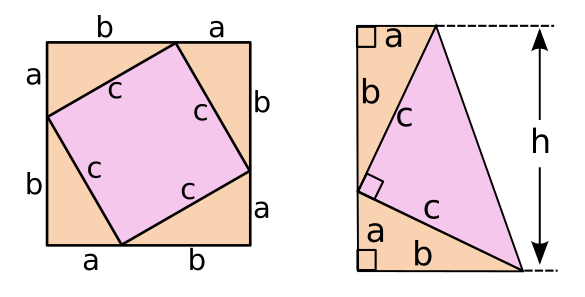Proofs of the Pythagorean theorem by Bhaskara (left) and U.S. President, James Garfield (right). In the Bhaskara proof, the area of the inner square is c2, the area of the outer square is (a+b)2, and the area of each triangle is (1/2)(ab). US President James Garfield's proof equates the area of the trapezoid to the combined area of the triangles. In each case, the formulas for the areas of the geometrical objects, plus a little math, gives the theorem. (Drawing by the author using Inkscape.)(Click for larger image.)

Armed with a compass and straightedge, I also tried my hand at trisecting angles. I was told that angle trisection was not possible; but, all it takes to spark the interest of a child is to tell him that it can't be done. Angle trisection had been proven to be impossible in 1837 by the French mathematician, Pierre Wantzel (1814-1848), but I didn't know the details of his proof. I don't understand his proof even today, and not merely because his paper is in French.

I finally discovered a promising method of attack using the technique of successive bisections. The logic of this approach is similar to the method for determining the circumference of a circle by approximating it with polygons with a greater and greater number of sides. As an example, if you bisect an angle, then bisect the bisections to the point at which you have 16 smaller angles, you find that 16 is very close to 3x5 = 15, so you can create three angles quite close to a third of the original angle. This tedious method wasn't the simple solution that I desired, so I abandoned this quest and continued learning my New Math.

This brings us to another compass and straightedge challenge, creating a square having the same area as a circle, known simply as squaring the circle. The impossibility of this is easier to understand, since it involves the transcendental irrationality of pi. However, as Indian mathematician, Srinivasa Ramanujan, showed in 1913, and again in 1914, it's possible to create geometrical constructions by compass and straightedge that come extremely close to getting the exact value of pi. In the 1914 case, shown below, this simple figure squares a circle with a value of pi = 3.1415926525826461252, a value that's accurate to eight decimal places.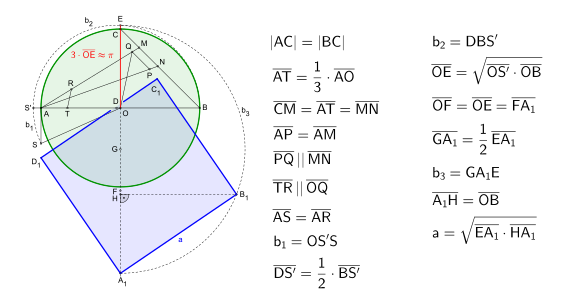Approximate squaring of a circle by Srinivasa Ramanujan. The squaring is quite close, since the pi value is accurate to eight decimal places. (Reformatted Wikimedia Commons image by Petrus3743. Click for larger image.)

The approximate squaring of the circle is still interesting to mathematicians. Hung Viet Chu, a doctoral candidate in mathematics at the University of Illinois at Urbana–Champaign (Urbana, Illinois), has just published a paper entitled, "Square the Circle in One Minute," on arXiv. In his paper, Chu reviews the previous efforts, including that of Ramanujan as shown above, and he improved the squaring with a method that uses an approximation of pi that's accurate to nine decimal places. Chu's expression for pi and its deviation from the actual value can be seen from the following equation:All the mathematical operations involved in this equation can be realized with a compass and straightedge. Addition is done by measuring line seqments with a compass and patching them together on a line. Multiplication and division can be done, also. An example multiplication is shown below.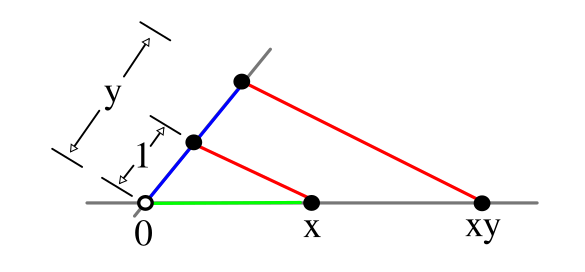Multiplication of two line seqments of length x and y proceeds by first orienting one of the segments at an arbitrary angle to the other, marking the unit length on one, and generating the parallel lines as shown. The construction is seen to be valid, since the property of similar triangles makes x/1 = xy/y, so xy = xy. (Created using Inkscape. See Wikibooks for details.)

The square-root of five in the equation is easily realized by a construction such as the creation of the golden ratio (φ), as shown below.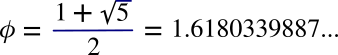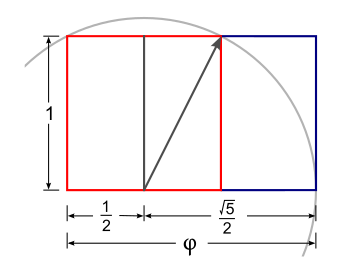Constructing the golden ratio (φ), and getting the square-root of five in the process.

First, draw a unit square (shown in red), then create a circle with an origin at half its width and a radius to a diagonal. Creating the rectangle, as shown, gives the golden ratio.

(Modified Wikimedia Commons image by Joel Holdsworth).

As my professors used to say after they demonstrated that a proof was possible, "the solution is left as an exercise for the student." As you can see from the several large numbers in the pi equation, this is not a simple exercise. The best explanation is in Chu's paper on arXiv.

References:

1. Elisha Scott Loomis, "The Pythagorean proposition: its demonstrations analyzed and classified, and bibliography of sources for data of the four kinds of proofs," Education Resources Information Center. Institute of Education Sciences (IES) of the U.S. Department of Education (1940); reprinted in 1968 by National Council of Teachers of Mathematics (310 page PDF File).
2. Franz Lemmermeyer, "On Leonardo da Vinci's proof of the Theorem of Pythagoras," arXiv, November 4, 2013.
3. XX P M L Wantzel, "Recherches sur les moyens de reconnaître si un problème de Géométrie peut se résoudre avec la règle et le compas," Journal de Mathématiques Pures et Appliquées, Series 1, Book 2 (1837), pp. 366-372 (PDF file).
4. S. Ramanujan, "Modular equations and approximations to π," Quarterly Journal of Mathematics, vol. XLV (1914), pp. 350-372.
5. Hung Viet Chu, "Square the Circle in One Minute," arXiv, August 3, 2019.
6. Descriptive Geometry/Mathematical Constructions/Multiplication/Division, Wiki Books.

Linked Keywords: Pythagorean theorem; elementary school; student; fundamental mathematics; Ancient Greek philosophy; Greek philosopher; Pythagoras; Pre-Socratic philosopher; Thales; whim; deity; god; models of scientific inquiry; scientific explanation; natural phenomenon; Thales' theorem; inscribed angle; semicircle; right angle; Ancient Greece; Greek; island; Samos; Anno Domini; BC; religious movement; number; fundamental reality; philosophy; string theory; string theorist; quotation; mathematics; universe; Wikimedia Commons; mathematical proof; 12th century; Hindu; mathematician; Bhaskara (1114-1185); President of the United States; James Garfield (1831-1881); United States House of Representatives; Leonardo da Vinci (1452-1519); German; physicist; Johannes Tobias Mayer (1752-1830); area; square; triangle; trapezoid; formula; geometry; geometrical; Inkscape; compass and straightedge; angle trisection; trisecting angles; child; France; French; Pierre Wantzel (1814-1848); academic publishing; paper; French language; successive bisection; logic; circumference; circle; approximation; approximating; polygon; bisect an angle; New Math; squaring the circle; transcendental number; irrationality of pi; India; Indian; Srinivasa Ramanujan; pi; decimal place; Hung Viet Chu; doctoral candidate; University of Illinois at Urbana–Champaign (Urbana, Illinois); arXiv; approximation error; deviation from the actual value; equation; mathematical operation; addition; line seqment; multiplication; division (mathematics); angle; unit vector; unit length; parallel (geometry); compass-and-straightedge construction; similar triangles; Inkscape; Wikibooks; square-root; golden ratio; unit square; origin (mathematics); radius; diagonal; professor.